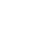# [Unity] 貝茲曲線假彈道製作原理與語法

## 線性貝茲曲線

t 代表時間，A 和 B 分別代表原點與終點，C 就是結果。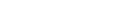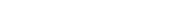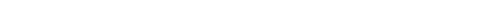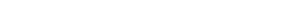## 二次方貝茲曲線

1. 先定義由左到右的座標分別為 A、B、C 點。
2. 分別計算 A~B 與 B~C 上的點於指定 t 的時候所在的座標，可得出的 D、E 兩個座標點。
3. 將 D 與 E 帶入公式最後得出我們要的 F 座標：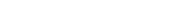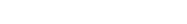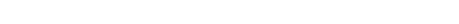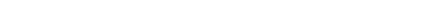### 二次方貝茲曲線公式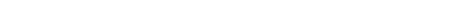## 語法

Unity 中的次方可使用 Mathf.Pow 來實現，最後的 Bt 座標就是指定時間點 t 的貝茲曲線座標。

public Transform P0, P1, P2, Bt;

private void Start()
{
var t = 0.5f;
var p0 = P0.position;
var p1 = P1.position;
var p2 = P2.position;
Bt.position = Mathf.Pow(1 - t, 2) * p0 + 2 * t * (1 - t) * p1 + Mathf.Pow(t, 2) * p2;
}

using UnityEngine;

public class BezierCalculator : MonoBehaviour
{
public int Duration = 5;
public Transform P0, P1, P2, Bt;

private float _time;
private float _duration;

private void Update()
{
if (_duration > Duration)
{
_duration = 0;
}

var t = _duration / Duration;
Bt.position =
Mathf.Pow(1 - t, 2) * P0.position +
2 * t * (1 - t) * P1.position +
Mathf.Pow(t, 2) * P2.position;

_duration += Time.deltaTime;
}
}


## 作者: